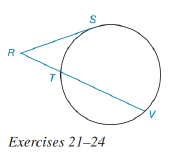Chapter 6.3, Problem 21E### Elementary Geometry for College St...

6th Edition
Daniel C. Alexander + 1 other
ISBN: 9781285195698

#### Solutions

Chapter
Section### Elementary Geometry for College St...

6th Edition
Daniel C. Alexander + 1 other
ISBN: 9781285195698
Textbook Problem
1 views

# In the figure for Exercises 21 to 24, R S ¯ is tangent to the circle at S. See Theorem 6.3.7.Given: R S = 8     a n d     R V = 12 Find: RT.To determine

To find:

To find RT.

Explanation

Given that, RS=8andRV=12.

The diagrammatic representation is given below,

Theorem:

If a tangent segment and a secant segment are drawn to a circle from an external point, then the square of the length of the tangent equals the product of the length of the secant with the length of its external segment.

By using the theorem to get the following,

RS2=RVRT

We know that

RV=RT+TV

Substitute the values RS=8

### Still sussing out bartleby?

Check out a sample textbook solution.

See a sample solution

#### The Solution to Your Study Problems

Bartleby provides explanations to thousands of textbook problems written by our experts, many with advanced degrees!

Get Started

#### Prove that abx2dx=b3a33.

Single Variable Calculus: Early Transcendentals, Volume I

#### limx6(x210x)=. a) 24 b) 6 c) 26 d) does not exist

Study Guide for Stewart's Single Variable Calculus: Early Transcendentals, 8th

#### A normal vector to the plane 2x + 3y + 6z =–20 is:

Study Guide for Stewart's Multivariable Calculus, 8th

#### Graph each function. fx=-5x+4

College Algebra (MindTap Course List)